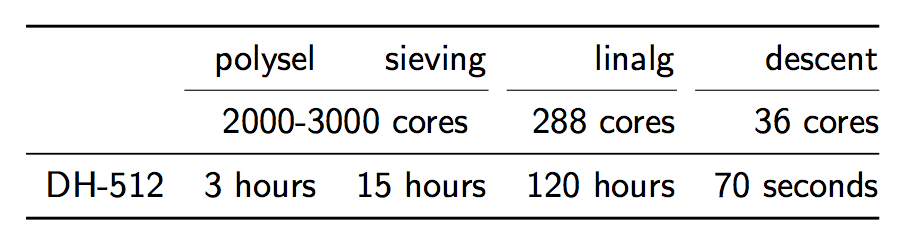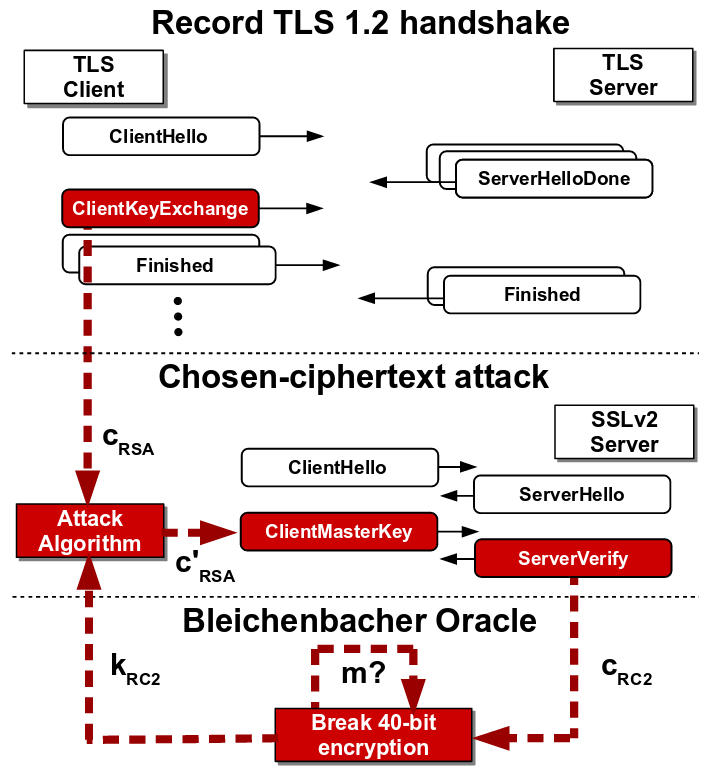Understanding and Securing TLS

· by Team Cinnamon

Last week we learned about Padding Oracle Attacks which use side-channel information related to ciphertext message padding in order to deduce the underlying plaintext message in a TLS communication. This week we will learn about Downgrade Attacks that force a TLS server to choose weaker encryption and protocol suit thereby making it vulnerable to attacks like man-in-the-middle.

We begin with a brief introduction of Diffie-Hellman Key Exchange protocol commonly used in TLS and then describe the Logjam attack on Diffie-Hellman protocol which relies on pre-computation of discrete-logs of a 512-bit prime used in the protocol. Next we discuss about State-level Threats to Diffie-Hellman, where we show that the pre-computation of discrete-logs is in the reach of current academic computation power. The practical consequence is that 8% of the top 1 million websites that use HTTPS can be broken in real time.

In the remainder of the article, we discuss about Bleichenbacher Attack which is a padding oracle attack on PKCS#1 v1.5 padding used in SSLv2. We conclude with Drown Attack that uses Bleichenbacker attack to gain access to RSA key of a TLS communication.

# Diffie-Hellman Cryptanalysis

Diffie-Hellman is a cryptologic method used to confidentially generate a shared secret (encryption key) between two parties in a conversation. Because the shared secret is used to encrypt message traffic, the integrity of Diffie-Hellman is crucial to the security of TLS, where the confidentiality of communication depends heavily on the process of securely generating a shared encryption key.

## Background: Diffie-Hellman Key Exchange

The genius of Diffie-Hellman lies in its adherence to the principle of perfect forward secrecy. The asymmetric keys used to perform the key exchange can be deleted after the key exchange is completed, so there is no risk of a later compromise enabling an attacker to break previous traffic. The agreed shared key is never transmitted, saved, or otherwise made observable across a communication channel, even if the adversary were to collect all encrypted data in transit and recover all material stored at the endpoints, it would still not be able to break the encryption.

The mathematical underpinnings of Diffie-Hellman are relatively simple. See the following image for an illustration of the exchange that occurs when generating a shared secret. Note that the secret values, a and b, are never transmitted nor shared between Alice and Bob.Image Source: https://i.stack.imgur.com/uYqQe.png

## The Diffie-Hellman Problem

The Diffie-Hellman problem (DHP) is as follows: Given an element $$g$$ and the values $$g^x, g^y$$ what is the value of $$g^{xy}$$?

Clearly, if this problem were easy to solve, Diffie-Hellman would be useless, since any eavesdropping adversary could then collect both $$g^x$$ and $$g^y$$, compute $$g^{xy}$$, and subsequently decrypt all of Alice and Bob’s communication. The Discrete Logarithm Problem (DLP) is thus by far the most efficient means to solve DHP and requires that the eavesdropping adversary compute $$x$$ given $$g$$ and $$g^x$$ – still believed to be a hard problem in general. But, if $$p$$ is a weak prime (as explained below), it can solved in a reasonable amount of time with available computing resources.

Adrian et al. published a paper in 2015 that demonstrated a weakness in Diffie-Hellman key exchange in the Handshake Protocol of TLS. In order to perform cryptanalysis in such a situation, an attacker must solve DLP by computing arbitrary discrete log values in real-time. While it is not known how to calculate discrete logs efficiently (and believed to be hard), the attack can be accelerated using precomputation.

## Index Calculus

While the logic behind the precomputation cryptanalysis is based on the General Number Field Sieve, we examine Index Calculus, a simplified version of the same concept. Index Calculus involves two steps: sieving and linear algebra. In the sieving step, the cryptanalyst chooses a multiplicative group of numbers and attempts to find numbers that factor completely over this group. We can then express these numbers as linear combinations of logs of the multiplicative group elements. By finding many such numbers, we obtain equations in terms of logs of multiplicative group elements. In the linear algebra step, we can use these equations to solve for values of the logs. This allows us to generate a database of precomputed logs.

Using precomputed discrete logs, an attacker can perform an active downgrade attack on a TLS connection. Operating as an in-the-middle attacker, the attacker intercepts a Client Hello message and alters it to instruct the server to use export-grade Diffie-Hellman, which uses 512-bit keys rather than 1024-bit or higher, which allows the attacker to use the precomputed logs. During the Handshake Protocol, the attacker is able to solve the discrete log problem and recover the master secret of that connection. This allows the attacker to communicate directly with the client over an encrypted channel, while the client still thinks he/she is communicating with their intended server.

## The Logjam Attack: Step-by-Step1. The Client sends a Client Hello message to the Server. This includes a list of all supported ciphersuites that can be used to generate the shared secret and requests that one be selected by the Server to begin the Diffie-Hellman exchange.

2. The adversary intercepts the Client Hello messages and alters the available ciphersuites to only include 512-bit Diffie-Hellman, then forwards the altered Client Hello to the Server.

3. The Server receives the Client Hello. Since only one ciphersuite is shown to be supported by the Client, the Server agrees to 512-bit Diffie-Hellman and generates a 512-bit prime $$p$$ to be used in the key exchange.

4. The adversary intercepts the ciphersuite confirmation message from the Server and alters it to reflect the client’s original ciphersuite preference, selecting full-strength DHE. It also eavesdrops on the Server’s Diffie-Hellman communication to discern $$g$$ and $$gb$$.

5. The Client thinks it has received the proper Diffie-Hellman information from the Server and begins its half of the Diffie-Hellman exchange. Note that the 512-bit prime $$p$$ is still considered a valid prime for 1024-bit DHE. Meanwhile, the adversary is hard at work calculating the discrete log of $$g^b \mod p_{512}$$. Multiple factors can contribute to reduce the time-complexity of this process.

6. Once the adversary has determined the value of $$b$$, the Client and adversary begin communicating as though the adversary is the Server.

The time-complexity of precomputation using the number field sieve is entirely dependent on the prime number used; as a result, any connections using the same prime can be quickly broken. The researchers behind Logjam detected that millions of HTTPS, SSH, and VPN servers use the same prime numbers for Diffie-Hellman key exchange, rendering supposedly-secure communications vulnerable to Logjam.

## Weak Primes

Consider a prime number $$p$$. In 1978, Pohlig and Hellman demonstrated that if all factors of $$p-1$$ are less than $$\log^c p$$, the problem of solving the discrete logarithm $$\mod p$$ is in $$P$$. In general terms, this means an attacker can greatly reduce the complexity of the discrete logarithm problem and thus conduct the Logjam attack in a shorter period of time. We’ll demonstrate with an example using a small prime.

Let $$p = 31$$ and $$g = 3$$. $$g$$ has order 30 → $$g^{30} = 1 \mod p$$

The factors of $$30 = 2 * 3 * 5$$ are too small for the prime $$p$$ to be secure, allowing an attacker to convert the discrete logarithm problem $$\mod 31$$ into problems $$\mod 2, 3, 5$$. With the help of the Chinese Remainder Theorem, an attacker can easily find $$x$$ given $$g$$ and $$g^x$$.

A strong prime $$p = 2q + 1$$ ($$q$$ is prime) avoids this problem of reducibility by ensuring that $$(p-1)\div 2$$ cannot be composite (and, by extension, that Pohlig-Hellman cannot obtain information from $$p$$).

This puts a server that supports export-grade DH and reuses weak primes at severe risk to a motivated attack.

# State-Level Threats to Diffie-Hellman

## Current Situation

In recent years, the general bar for internet security has raised substantially. HTTPS is now the norm for most heavily trafficked websites and is spreading through services such as Let’s Encrypt, that allow for domain owners to easily get SSL certificates for a website at no cost. In general, this trend looks positive: for key exchange protocols, stronger 768 and 1024-bit groups are now the norm rather than the exception. Individual users and institutions have also begun to better understand the need for security and IPSec Virtual Private Networks (VPNs) and SSH connects are being more broadly practiced.

While standards have advanced and security has increased in general, cryptanalysis techniques and computational power have also increased. Although ideas such as Moore’s Law, that computing power at a certain price level effectively doubles every 18 months, the practical implications are not as often taken into account. These days, DH-512 is easily within the reach of “academic power,” that is to say, within the reach of institutions with access to midsize computing facilities or individuals who can afford a few hundred thousand dollars of computing.

With recent hardware, to “crack” DH-512 takes 2.5 core-years in the sieving phase, 7.7 core-years in the linear algebra phase, but only 10 core-minutes in the descent phase. So, given a 2000-3000 core cluster, all of the phases combined except the descent phase takes about 140 hours.https://weakdh.org/weakdh-ccs-slides.pdf>

However, the descent phase only takes about 70 seconds. What does this mean? That after 1 week of pre-computation, an individual calculation can be completed in about 70s. If this is combined with something called a “downgrade attack,” which will be described below, connections to about 8% of top 1 million sites that use HTTPS can be broken in real time.

## DROWN Attack

DROWN attack requires that a TLS server and a SSLv2 server share an RSA key. The attacker records multiple TLS handshake messages between a client and the TLS server. The aim of the attacker is to decrypt the RSA key of the TLS handshake. To do so, the attacker forces the client to establish a connection with an SSLv2 server having the same RSA key so that the attacker can initiate the Bleichenbacher attack to recover the RSA key.

Now the main hurdle for the attacker is that the format of TLS handshake message may not comply with PKCS#1 v1.5 padding scheme of SSLv2. The attacker converts the TLS ciphertext to SSLv2 ciphertext using the concept of trimmers introduced by Bardou et al. which reduces the size of the TLS message. The use of trimmers require repeated querying to SSLv2 server by shifting the message bytes. The recovered SSLv2 plaintext is then converted back to TLS plaintext which reveals the RSA key of TLS handshake.SSLv2-based Bleichenbacher attack on TLS
Source: https://tlseminar.github.io/docs/drown.pdf

## Special DROWN Attack

The OpenSSL implementation had two bugs which led to a more efficient Bleichenbacher attack on an OpenSSL implementation of SSLv2 server.

OpenSSL extra clear oracle: OpenSSL implementation allowed non-export cipher messages to contain clear_key_data which lead to potential overwriting of key bytes with null bytes. An attacker could vary the number of null bytes to decrypt the whole key one byte at a time.

OpenSSL leaky export oracle: OpenSSL in export cipher mode allowed valid oracle response for correctly padded message of ‘any’ length.

These bugs remained in OpenSSL implementation from 1998 up until its patch in 2015, when the authors of DROWN contacted the OpenSSL developers.

## Prevention of DROWN

The attack is successful mainly because of the reliance on obsolete cryptographic practices. Export-grade ciphers only support 40-bit keys which are vulnerable to brute-force attack and hence it is crucial to disable export-grade ciphers and use safer ciphers (like AES_GCM) with longer key lengths (256-bits). PKCS#1 v1.5 padding leaks significant byte patterns and hence a better padding technique should be used. SSLv2 protocol includes the above obsolete cryptos and hence it should be scrapped and replaced with TLS 1.3. Lastly, the RSA public keys should not be shared among multiple servers or connections in order to deter the attack.